# Part 5: Non-Linear Relationships (Curve Sketching) | Free Worksheet

In this article, we give you a comprehensive breakdown of non-linear equations. We explain how these equations work and then illustrate how they should appear when graphed.## Non-linear relationships and curve sketching

Mastering Non-Linear Relationships in Year 10 is a crucial gateway to being able to successfully navigate through senior mathematics and secure your fundamentals. Here is our guide to ensuring your success with some tips that you should check out before going on to Year 10.

## NSW Syllabus Outcome

• Graph a variety of parabolas, including where the equation is given in the form $$y=ax^2+bx+c$$, for various values of $$a, b$$ and $$c$$
• Graph a variety of hyperbolic curves, including where the equation is given in the form $$y=\frac{k}{x}+c$$ or $$y=\frac{k}{x−a}$$ for integer values of $$k, a$$ and $$c$$
• Establish the equation of the circle with centre $$(a,b)$$ and radius $$r$$, and graph equations of the form $$(x−a)^2+(y−b)^2=r^2$$ (Communicating, Reasoning)
• Describe, interpret and sketch cubics, other curves and their transformations

## Outline

We will be covering the following topics:

• Non-linear relationships
• Curve sketching – Four types:
• Parabolas
• Cubics
• Hyperbolas
• Circles

## Assumed knowledge

Students should be familiar with the coordinate system on the cartesian plane.

They should understand the significance of common features on graphs, such as the $$x$$ and $$y$$ intercepts.

## Want to see how well you know Curve Sketching and Non-Linear Relationships?## Parabolas

Students should know how to solve quadratic equations in the form $$ax^2+bx+c$$ and put them in the completed square form $$y=(x+a)^2 +c$$.

From here, we should be able to sketch any parabola.

### $$y=x^2$$ | The Basic Parabola $$y=x^2$$

This is the most basic form of the parabola and is the starting point to sketching all other parabolas.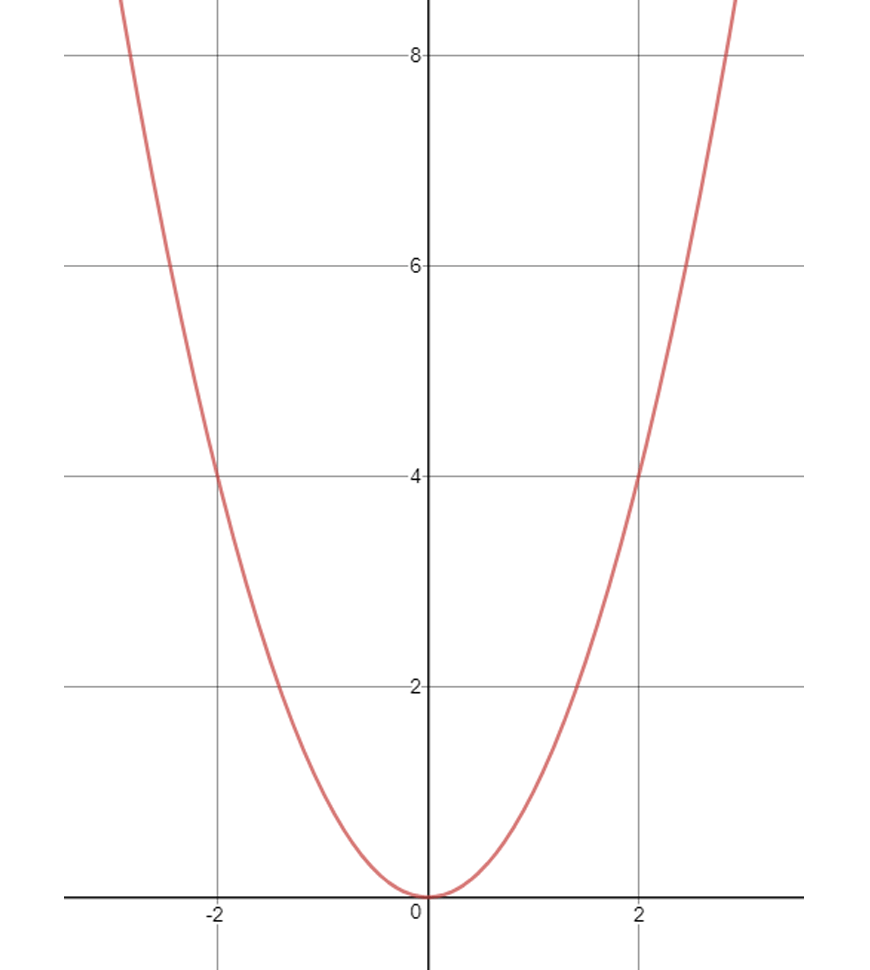In a parabola, there are two important details that we need to note down:

• The coordinates of the vertex
• The direction the parabola goes

For the most basic parabola as seen above, the vertex is at $$(0,0)$$, and the direction is upwards.

Now we will investigate the number of different transformations we can apply to our basic parabola.

### $$y=ax^2$$ | Scaling

The most basic transformation is a scaling transformation, which is denoted by a constant a being multiplied in front of the $$x^2$$ term.

The bigger the constant, the steeper the parabola. Let’s look at the graph $$y=3x^2$$.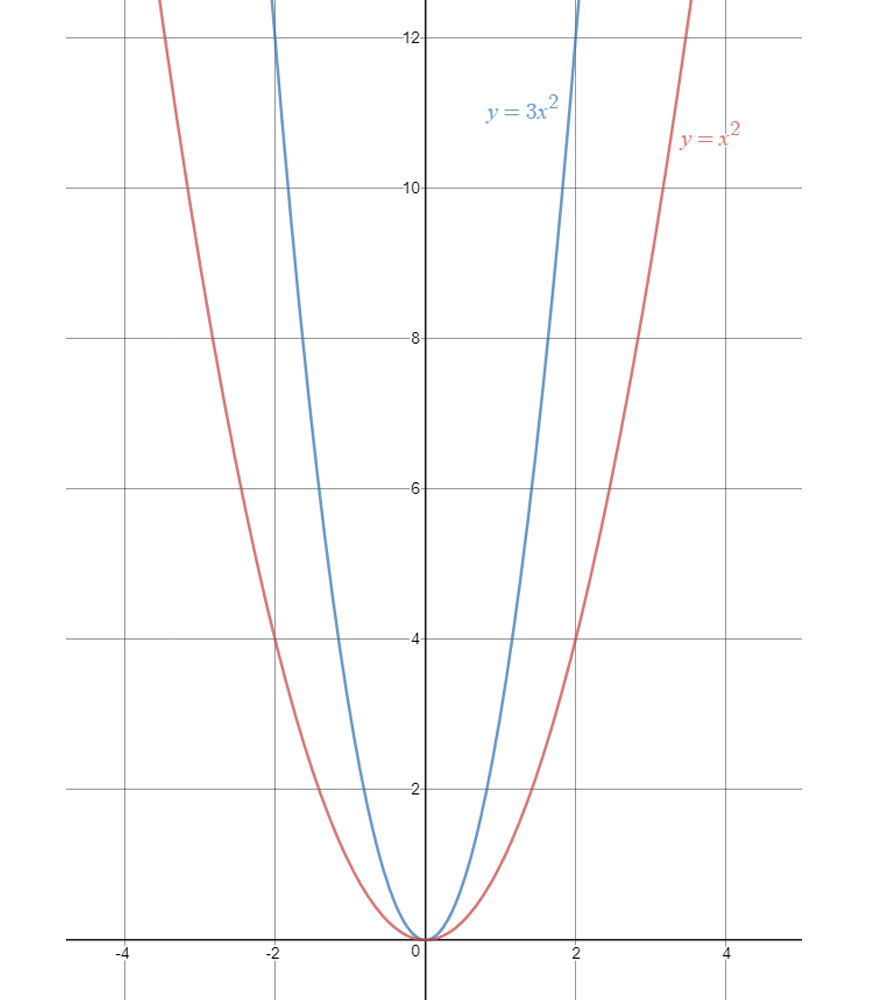Compare the blue curve $$y=3x^2$$ with the red curve $$y=x^2$$, and we can clearly see the blue curve is steeper, as it has a greater scaling constant $$a$$.

It is also important to note that neither the vertex nor the direction have changed.

### $$y=-x^2$$ | Negative Transformation

When we have a minus sign in front of the $$x^2$$, the direction of the parabola changes from upwards to downwards.

We can also say that we are reflecting about the $$x$$-axis.

Let’s look at the graph of $$y=-x^2$$.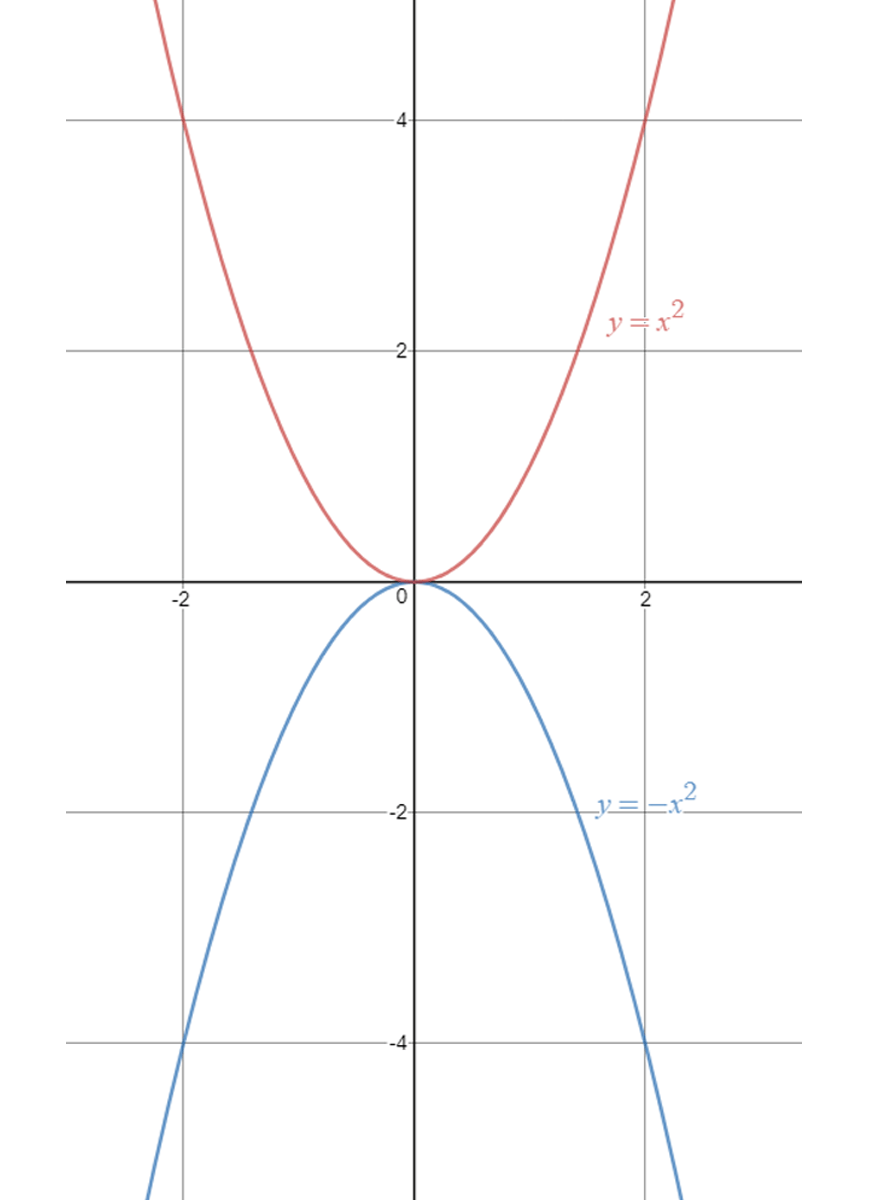Here we can clearly see the effect of the minus sign in front of the $$x^2$$.

The direction has changed, but the vertex has not.

### $$y=(x+a)^2$$ | Horizontal Shift

Now we will investigate changes to the vertex.

If we add a constant to the inside of the square, we are instigating a horizontal shift of the curve.

If this constant is positive, we shift to the left. Similarly if the constant is negative, we shift to the right.

For example, let’s take a look at the graphs of $$y=(x-3)^2$$ and $$y=(x+2)^2$$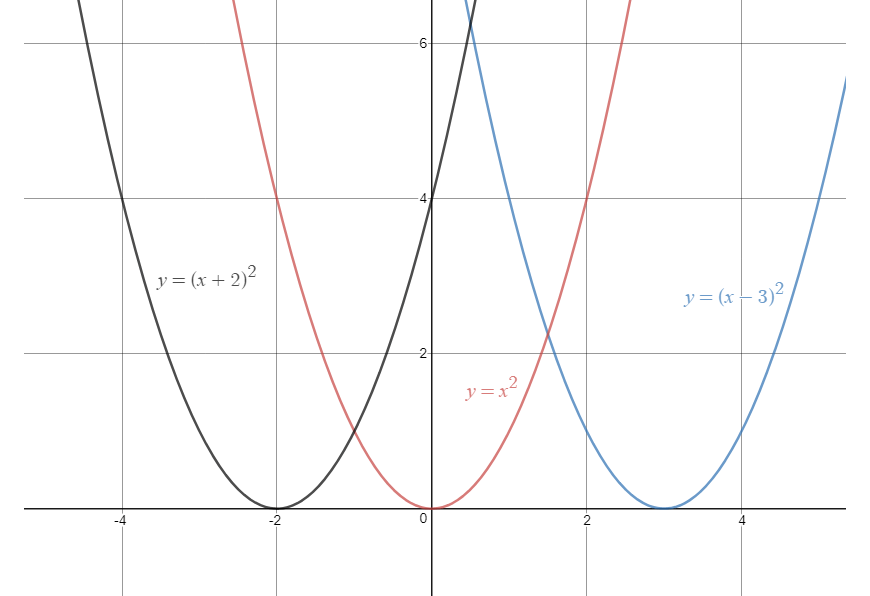The graph looks a little messy, but we just need to pay attention to the vertex of each graph.

We can see in the black curve $$y=(x+2)^2$$, the vertex has shifted to the left by $$2$$, dictated by the $$+2$$ in our equation.

Similarly, in the blue curve $$y=(x-3)^2$$, the vertex has shifted to the right by $$3$$, dictated by the $$-3$$ in our equation.

The direction of all the parabolas has not changed.

### $$y=x^2+c$$ | Vertical Shift

The final transformation is another shift in the vertex.

This time, we are instigating a vertical shift, dictated by adding a constant $$c$$ outside of the square.

Notice the difference from the previous section, where the constant was inside the square.

Here, if the constant is positive, we shift the vertex up.

Similarly, if the constant is negative, we shift the vertex down.

Take a look at the following graphs, $$y=x^2+3$$ and $$y=x^2-2$$.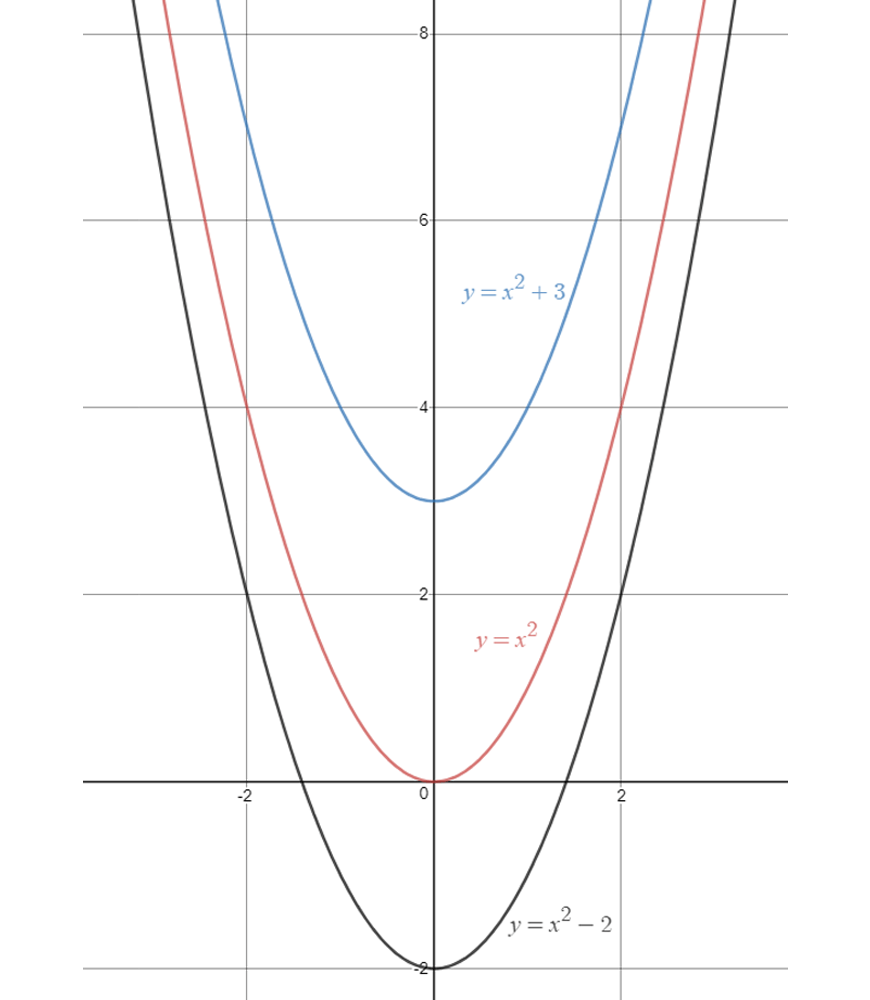Again, pay close attention to the vertex of each parabola.

In the black curve $$y=x^2-2$$, the vertex has been shifted down by $$2$$.

In the blue curve $$y=x^2+3$$, the vertex has been shifted up by $$3$$.

Again, the direction of the parabolas has not changed.

### Combining transformations

Remember that there are two important features of a parabola: vertex and direction.

By default, we should always start at a standard parabola $$y=x^2$$ with vertex $$(0,0)$$ and direction upwards.

We can then start applying the transformations we just learned.

Take a look at the following examples.

1.  $$y=(x-3)^2+5$$

Here, we can see two transformations.

We need to shift the vertex to the right by $$3$$ and up by $$5$$.

Remember, the constant inside dictates a horizontal shift.

The constant outside dictates a vertical shift.

Since there is no minus sign outside the $$(x-3)^2$$, the direction is upwards.

Therefore we have a vertex of $$(3,5)$$ and a direction upwards, which is all we need to sketch the parabola.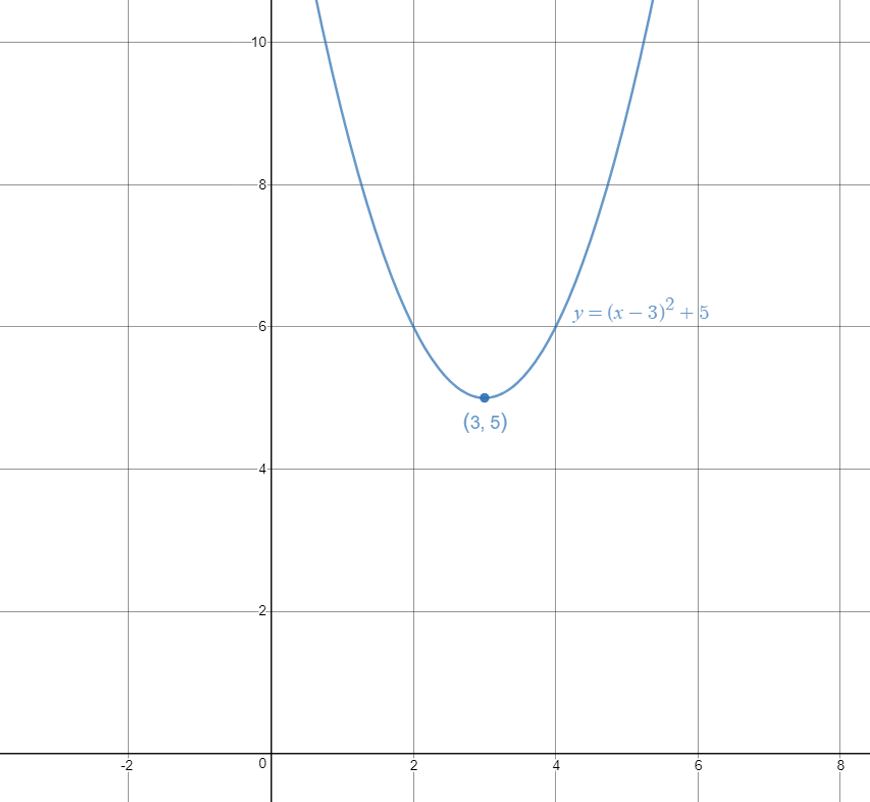2.  $$y=-x^2+3$$

To sketch this parabola, we again must look at which transformations we need to apply.

Since there is no constant inside the square, there is no horizontal shift.

However, there is a constant outside the square, so we have a vertical shift upwards by $$3$$.

So now we know the vertex should only be shifted up by $$3$$.

We also see a minus sign in front of the $$x^2$$, which means the direction of the parabola is now downwards.

Therefore we have a vertex $$(0,3)$$ and direction downwards.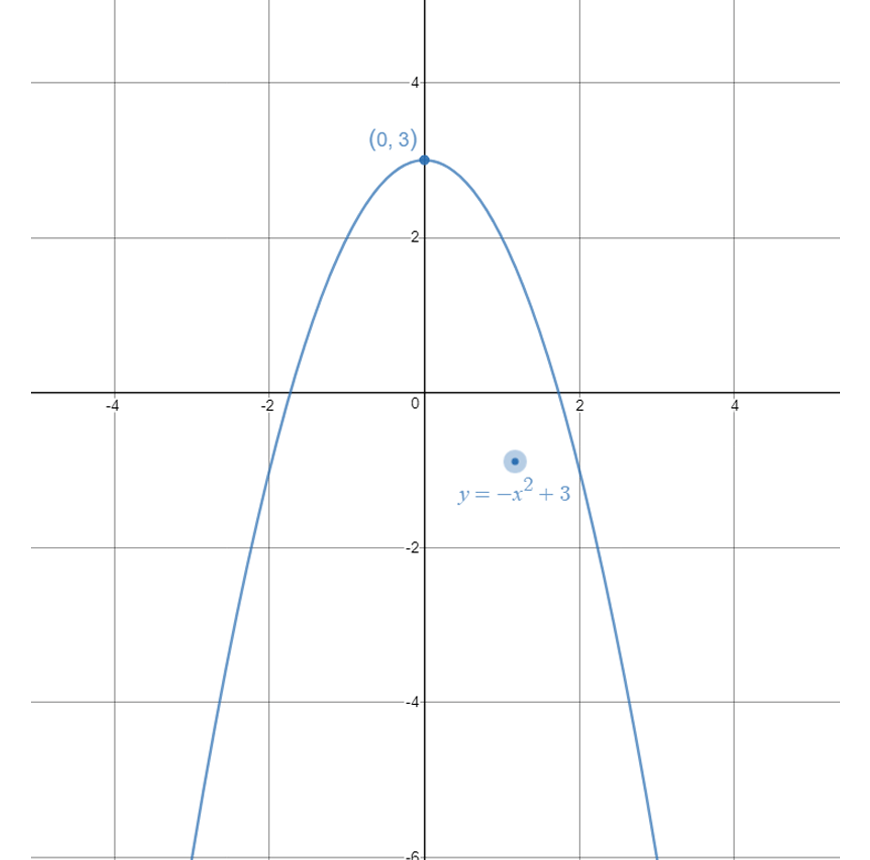Important note!

The transformations you have just learnt in parts 1-5 can be applied to any graph, not just parabolas! In the next sections, you will learn how to apply them to cubics, hyperbolas, and circles.

## Want to ace your next Maths exam?

With our Matrix Year 10 Maths Term Course, you will revise over core Maths topics, sharpen your skills and build confidence. Learn more now!## Cubics

Students should be familiar with the completed cubic form $$y=(x+a)^3 +c$$.

From here, we should be able to sketch any cubic, in very similar fashion to sketching parabolas.

### $$y=x^3$$ | The Basic Cubic

This is the most basic form of a cubic.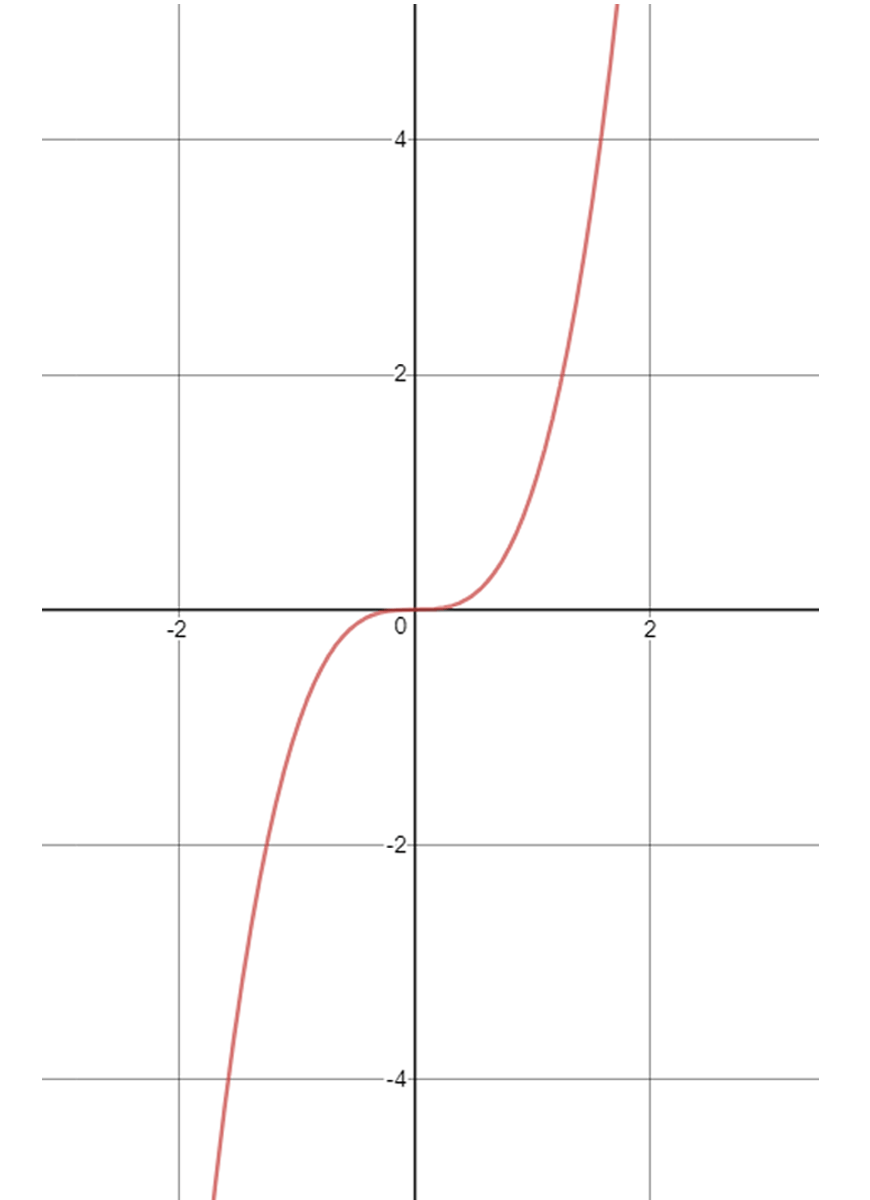In a cubic, there are two important details that we need to note down:

• The coordinates of the point of inflexion (POI)
• The direction the cubic goes.

Note this is extremely similar to a parabola, however instead of a vertex we now have a point of inflexion.

For the most basic cubic as seen above, the POI is at $$(0,0)$$, and the direction is from bottom-left to top-right, which we will call positive.

The transformations we can make on the cubic are exactly the same as the parabola.

We can shift the POI vertically or horizontally, and we can change the direction.

### $$y=ax^3$$ | Scaling

Again, we can apply a scaling transformation, which is denoted by a constant a being multiplied in front of the $$x^3$$ term.

The bigger the constant, the steeper the cubic.

Lets look at the graph $$y=4x^3$$.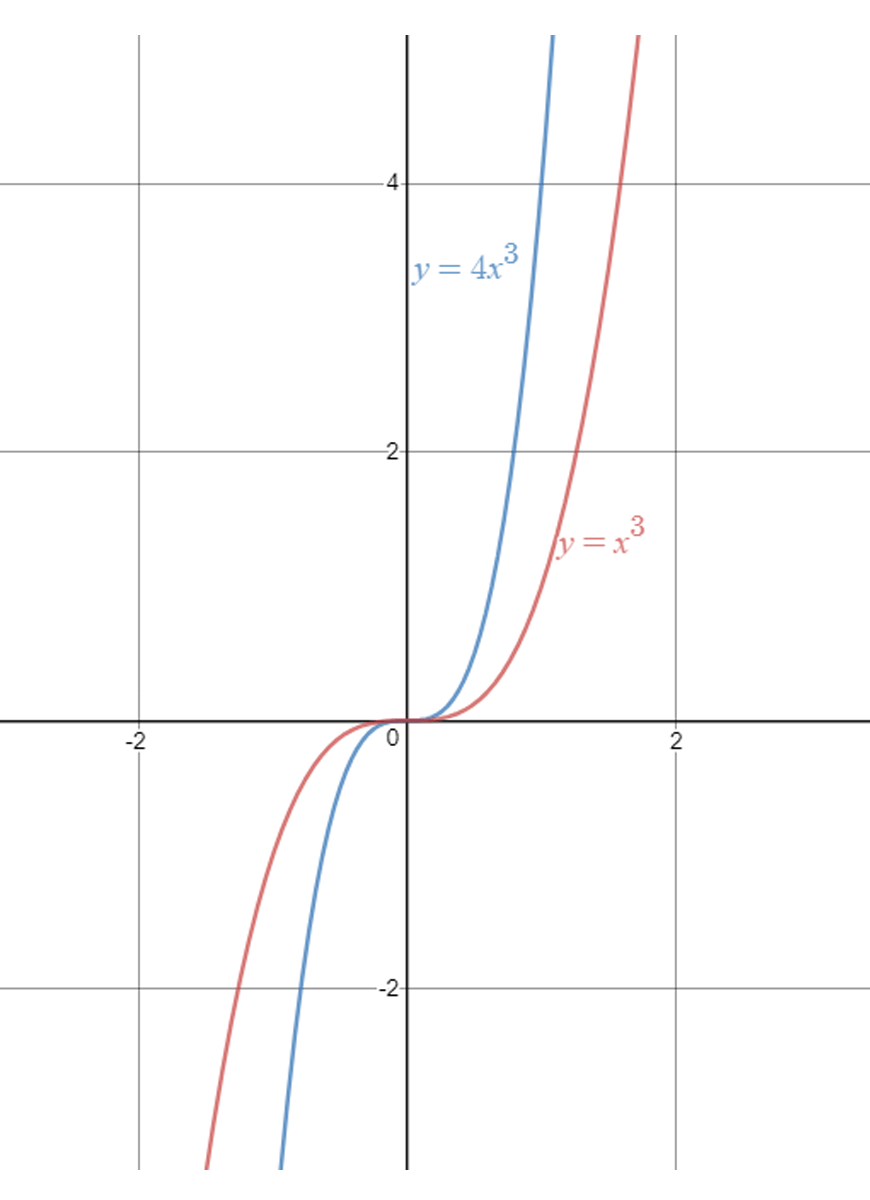Compare the blue curve $$y=4x^3$$ with the red curve $$y=x^3$$, and we can clearly see the blue curve is steeper, as it has a greater scaling constant $$a$$.

Again, similarly to parabolas, it is important to note that neither the POI nor the direction have changed.

### $$y=-x^3$$ | Negative Transformation

When we have a minus sign in front of the $$x^3$$, the direction of the cubic changes.

We can also say that we are reflecting about the $$x$$-axis. Let’s look at the graph of $$y=-x^3$$.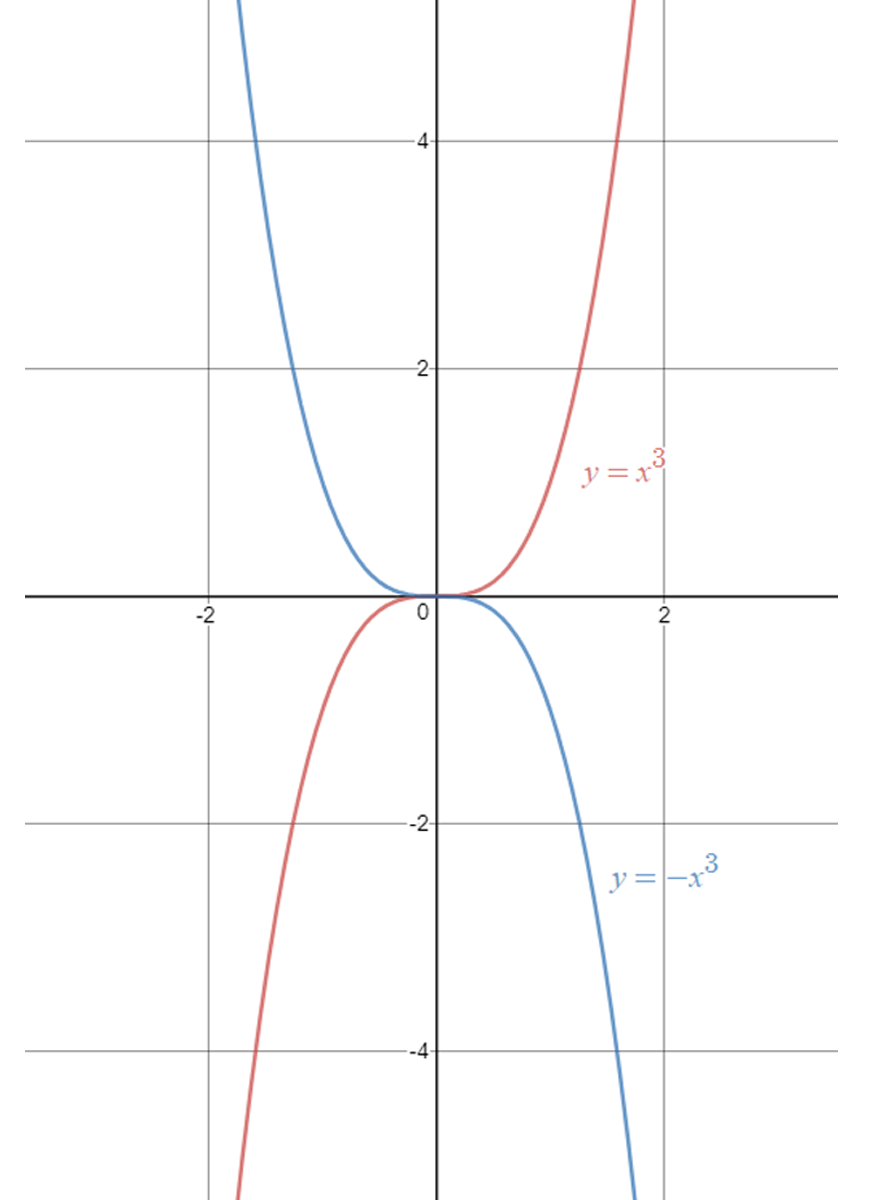Notice how the red curve $$y=x^3$$ goes from bottom-left to top-right, which is what we call the positive direction.

The blue curve $$y=-x^3$$ goes from top-left to bottom-right, which is the negative direction.

Also, in both curves, the point of inflexion has not changed from $$(0,0)$$.

### $$y=(x+a)^3$$ | Horizontal Shift

Now we will investigate changes to the point of inflexion (POI).

This, again, is very similar to a shift in a parabola’s vertex.

If we add a constant to the inside of the cube, we are instigating a horizontal shift of the curve.

If this constant is positive, we shift to the left.

Similarly if the constant is negative, we shift to the right. For example, let’s take a look at the graphs of $$y=(x+3)^3$$ and $$y=(x-2)^3$$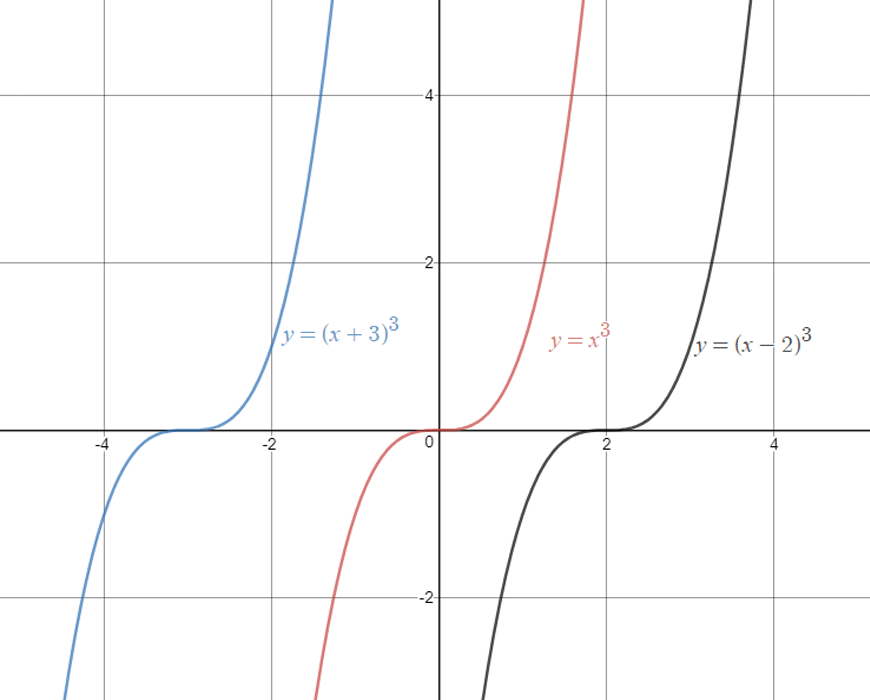Pay attention to the POI of each graph.

We can see in the black curve $$y=(x+2)^3$$, the vertex has shifted to the left by $$2$$.

Similarly, in the blue curve $$y=(x-3)^3$$, the vertex has shifted to the right by $$3$$.

We can see this is very similar to the horizontal shifting of parabolas.

The direction of all the cubics has not changed.

### $$y=x^3+c$$ | Vertical Shift

Finally, we investigate a vertical shift in the POI, dictated by adding a constant $$c$$ outside of the cube.

Notice the difference from the previous section, where the constant was inside the cube.

Here, if the constant is positive, we shift the POI up.

Similarly, if the constant is negative, we shift the POI down.

Take a look at the following graphs, $$y=x^3+3$$ and $$y=x^3-2$$.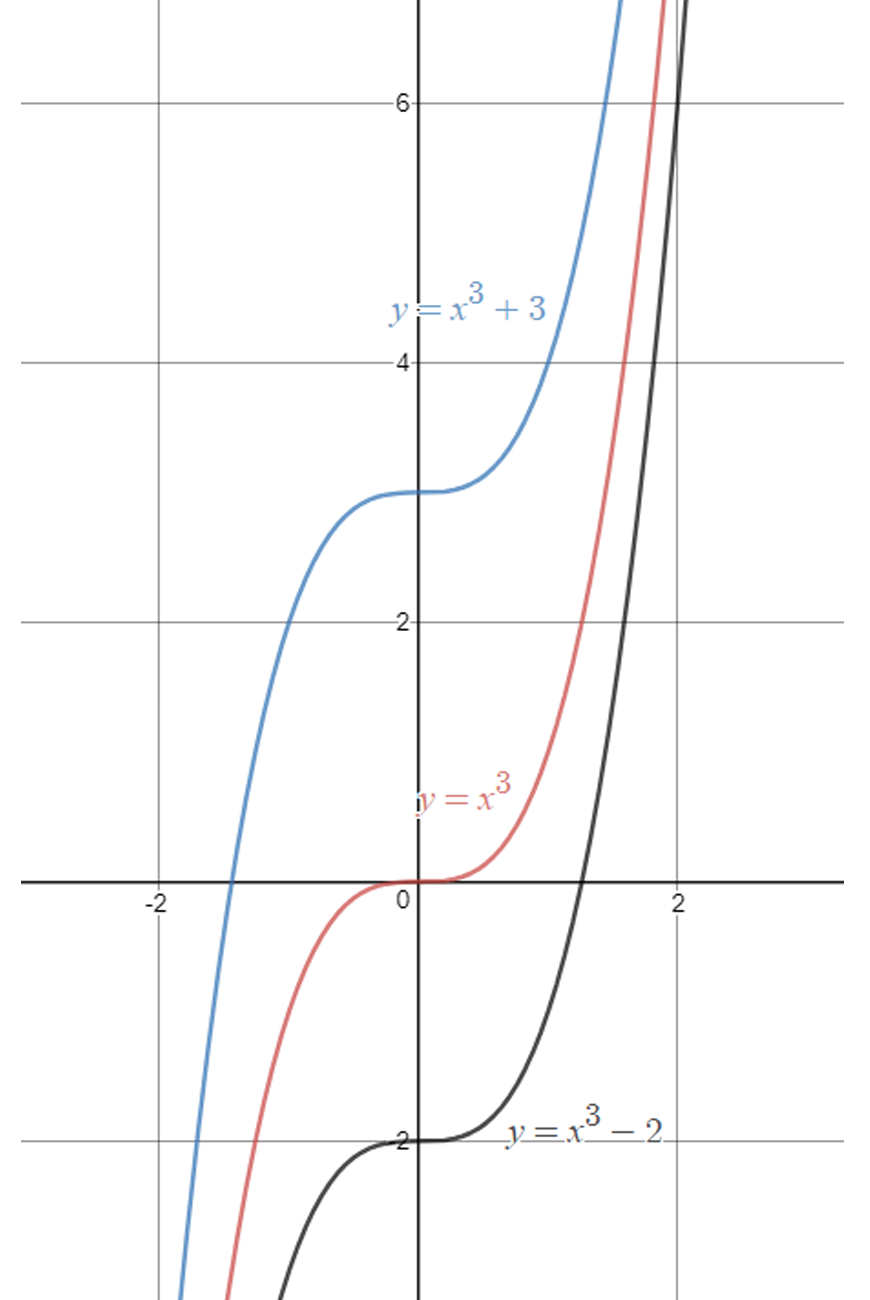Again, pay close attention to the POI of each cubic.

In the black curve $$y=x^3-2$$, the POI has been shifted down by $$2$$.

In the blue curve $$y=x^3+3$$, the vertex has been shifted up by $$3$$.

Again, the direction of the cubics has not changed.

### Combining transformations

Remember that there are two important features of a cubic: POI and direction.

By default, we should always start at a standard parabola $$y=x^3$$ with POI (0,0) and direction positive.

We can then start applying the transformations we just learned.

Take a look at the following example.

1.  $$y=(x+3)^3-5$$

Here, we can see two transformations.

We need to shift the POI to the left by $$3$$ and down by $$5$$.

Remember, the constant inside dictates a horizontal shift.

The constant outside dictates a vertical shift.

Since there is no minus sign outside the $$(x+3)^3$$, the direction is positive (bottom-left to top-right).

Therefore we have a POI of $$(-3,-5)$$ and a direction positive, which is all we need to sketch the cubic.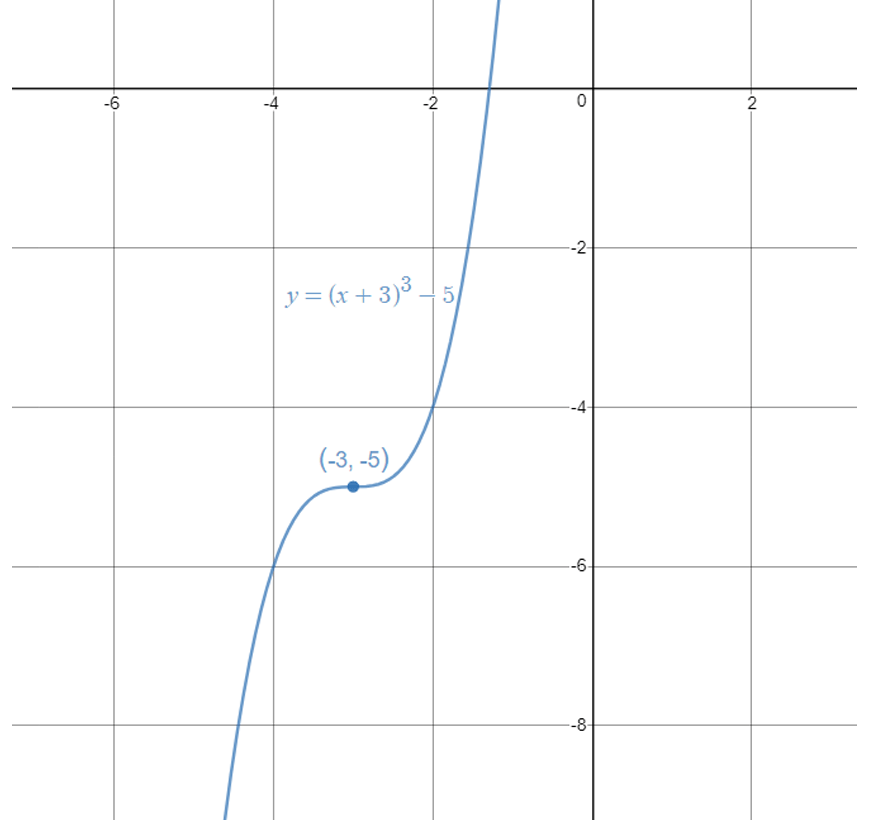## Hyperbolas

### $$y=\frac{1}{x}$$ | The Basic Hyperbola

This is the most basic form of a hyperbola.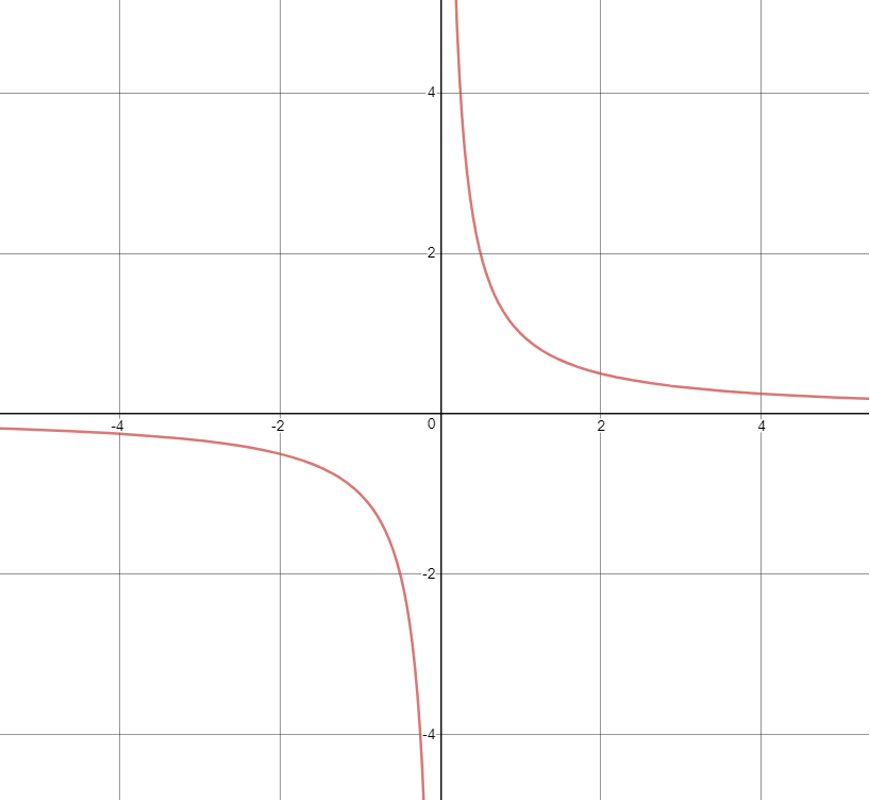Hyperbolas are a little different from parabolas or cubics.

Instead of a vertex or POI, hyperbolas are constricted into quadrants by vertical and horizontal asymptotes.

For the basic hyperbola, the asymptotes are at $$x=0$$ and $$y=0$$, which are also the coordinate axes.

Hyperbolas have a “direction” as well, which just dictates which quadrants the hyperbola lies in.

For the positive hyperbola, it lies in the first and third quadrants, as seen above.

### $$y=\frac{a}{x}$$ | Scaling

Again, we can apply a scaling transformation, which is denoted by a constant $$a$$ in the numerator.

The bigger the constant, the “further away” the hyperbola. This difference is easily seen by comparing with the curve $$y=\frac{2}{x}$$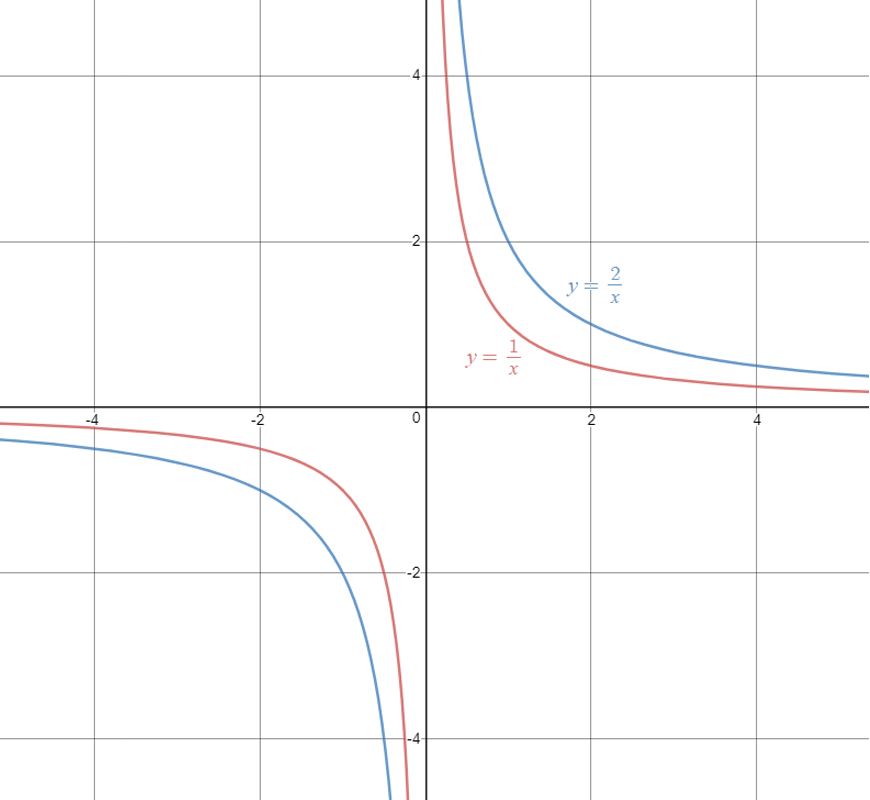Compare the blue curve $$y=\frac{2}{x}$$ with the red curve $$y=\frac{1}{x}$$, and we can clearly see the blue curve is further from the origin, as it has a greater scaling constant $$a$$.

However, notice that the asymptotes which define the quadrants have not changed.

### $$y=-\frac{1}{x}$$  | Negative Transformation

When we have a minus sign in front of the x in front of the fraction, the direction of the hyperbola changes.

Simply, a negative hyperbola occupies the second and fourth quadrants.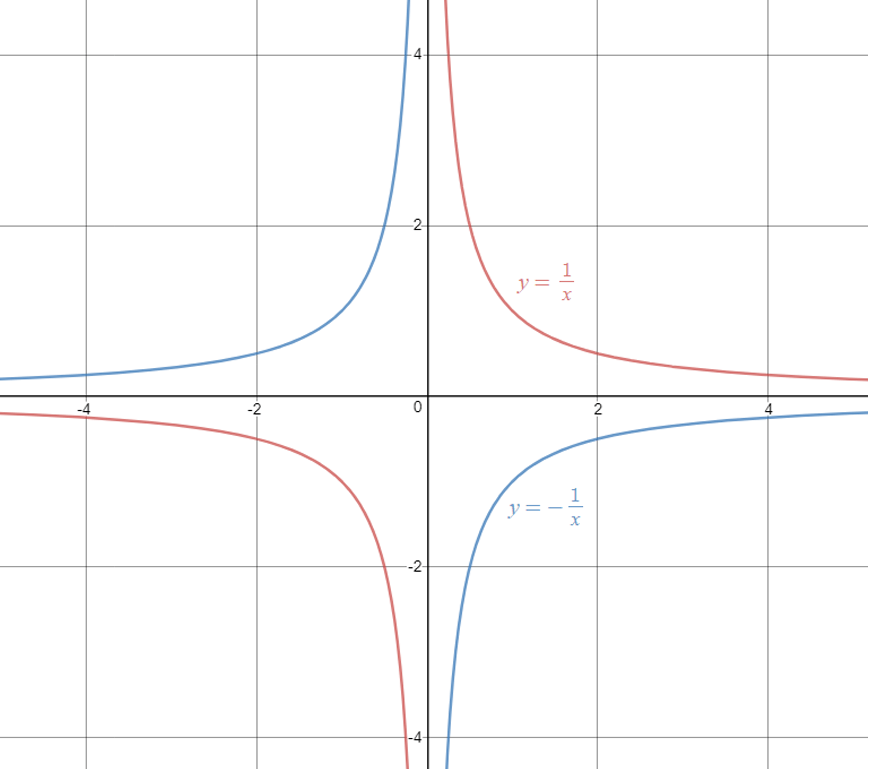Notice how the red curve $$y= \frac{1}{x}$$ occupies the first and third quadrants. This is what we call a positive hyperbola.

The blue curve $$y=-\frac{1}{x}$$ occupies the second and fourth quadrants, which is a negative parabola.

The only thing to remember here is that if there is a minus sign in front of the fraction (or if the equation can be manipulated in that form), it is a negative hyperbola.

### $$y=\frac{1}{(x+a)}$$ | Horizontal Shift

Now we will investigate horizontal shifting of a hyperbola.

If we add a constant inside the denominator, we are instigating a horizontal shift of the curve.

If this constant is positive, we shift to the left. Here, we should be focusing on the asymptotes.

When we shift horizontally, we are really shifting the vertical asymptote.

Similarly if the constant is negative, we shift to the right.

For example, let’s take a look at the graph of $$y=\frac{1}{(x+3)}$$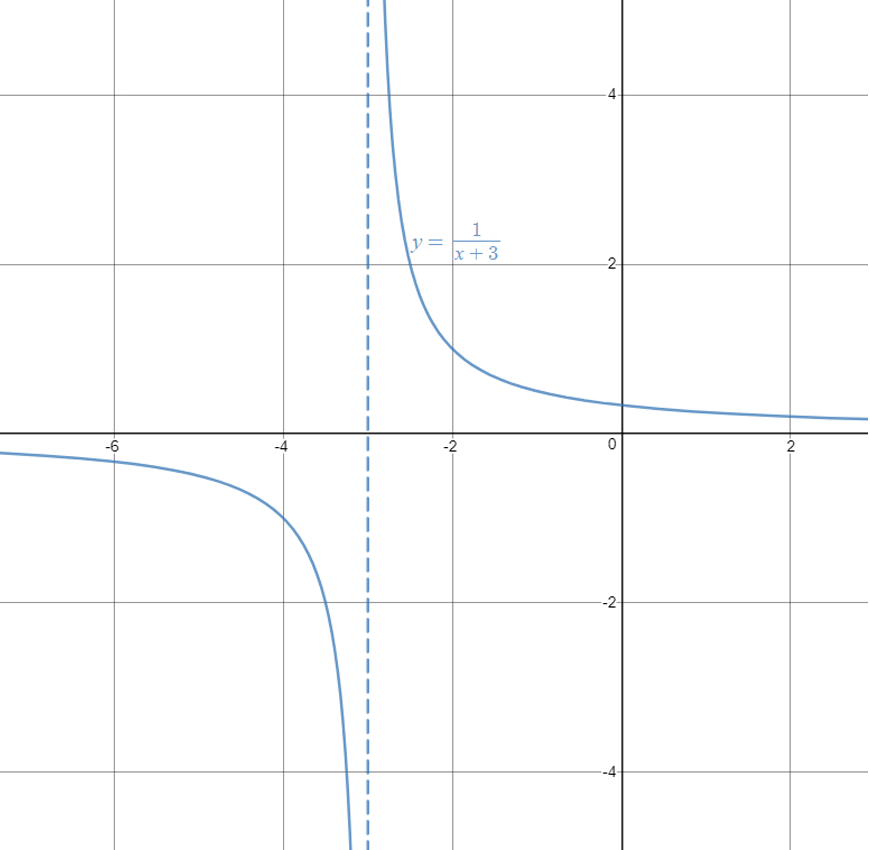We can see the hyperbola has shifted left by $$3$$.

A better way of looking at it is by paying attention to the vertical asymptote.

The vertical asymptote has shifted from the $$y$$-axis to the line $$x=-3$$ (ie. a left shift of 3 units).

This new vertical asymptote, alongside the horizontal asymptote $$y=0$$ (which has not changed), dictate where the quadrants are on the plane.

Since there is no minus sign in front of the fraction, the hyperbola is positive and lies in the first and third quadrants

### $$y=\frac{1}{x}+c$$ | Vertical Shift

Finally, we investigate a vertical shift in the hyperbola, dictated by adding a constant $$c$$ outside of the fraction.

Notice the difference from the previous section, where the constant was inside the denominator.

Here, if the constant is positive, we shift the horizontal asymptote up.

Similarly, if the constant is negative, we shift the horizontal asymptote down. Take a look at the following graph $$y=\frac{1}{x}+3$$.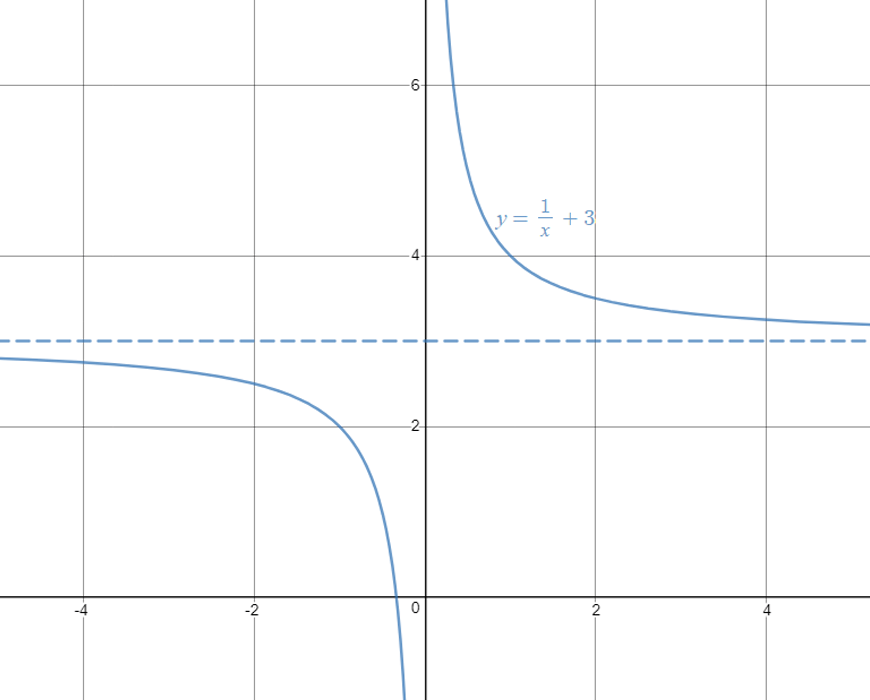We can see now that the horizontal asymptote has been shifted up by $$3$$, while the vertical asymptote has not changed at $$x=0$$.

These new asymptotes now dictate the new quadrants.

Since there is no minus sign in front of the fraction, the hyperbola lies in the first and third quadrants.

### Combining transformations

Remember that there are two important features of a hyperbola:

• Asymptotes
• The quadrants that it lies in

By default, we should always start at a standard parabola $$y=\frac{1}{x}$$ with coordinate axes as asymptotes and in the first and third quadrants.

We can then start applying the transformations we just learned.

Take a look at the following examples.

1. $$y=\frac{1}{(x-2)}+4$$

Here, we can see two transformations.

We need to shift the curve to the right by $$2$$ and up by $$4$$.

This means we need to shift the vertical asymptote to the right by $$2$$, and the horizontal asymptote upwards by $$4$$.

Since there is no minus sign in front of the fraction, the hyperbola lies in the first and third quadrants.

This is enough information to sketch the hyperbola.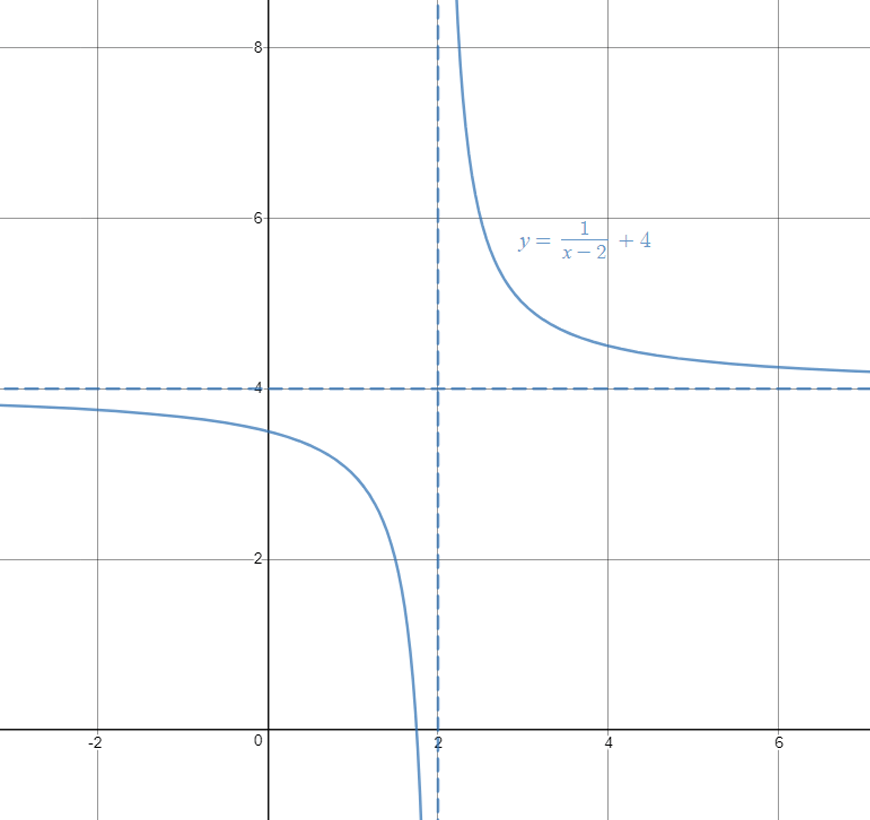2. $$y= \frac{1}{(4-x)}$$

Sometimes, it is easier to sketch a curve by first manipulating the expression, so we can draw features from it more clearly.

Generally, if there is a minus sign in front of the $$x$$, we should take out $$-1$$ from the denominator and put it in front of the fraction.

So, we can rewrite the equation as $$y=-\frac{1}{(x-4)}$$.

Now we can clearly see that there is a horizontal shift to the right by $$4$$.

There is also a minus sign in front of the fraction, so the hyperbola should lie in the second and fourth quadrants.

There is also no vertical shift.

This is now enough information to sketch the hyperbola.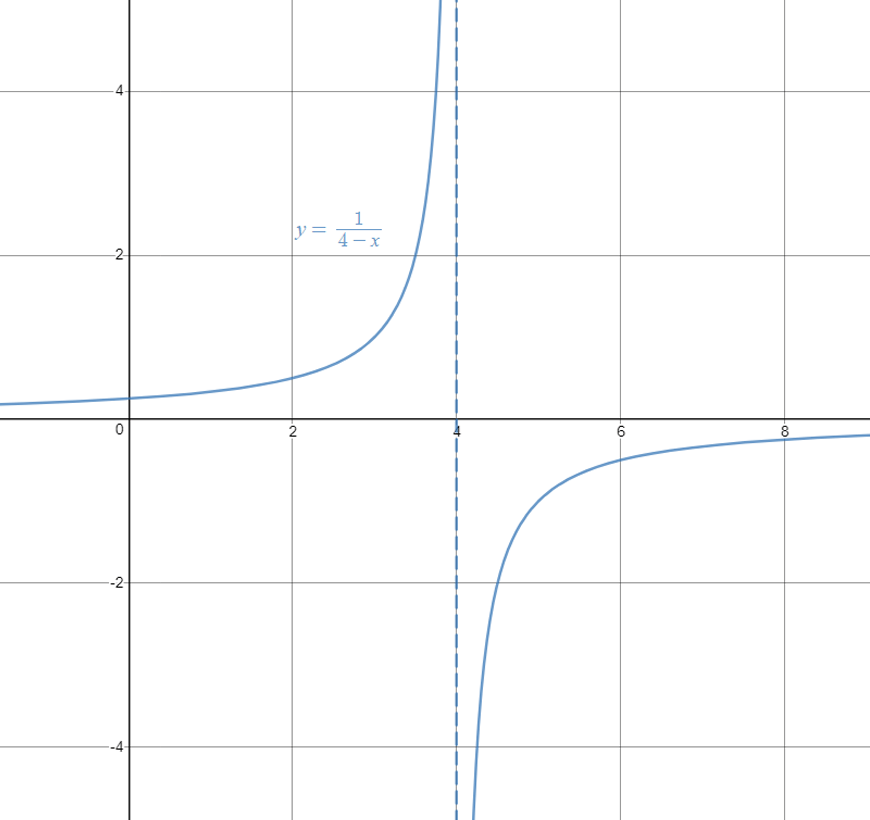## Circles

### $$x^2+y^2=r^2$$ | The Basic Circle

Circles are one of the simplest relations to sketch.

They have two properties: centre and radius.

The most basic circle has centre $$(0,0)$$ and radius $$r$$.

Note that if the term on the RHS is given as a number, we should first square root the number to find the actual radius, before sketching.

Take a look at the circle $$x^2+y^2=16$$.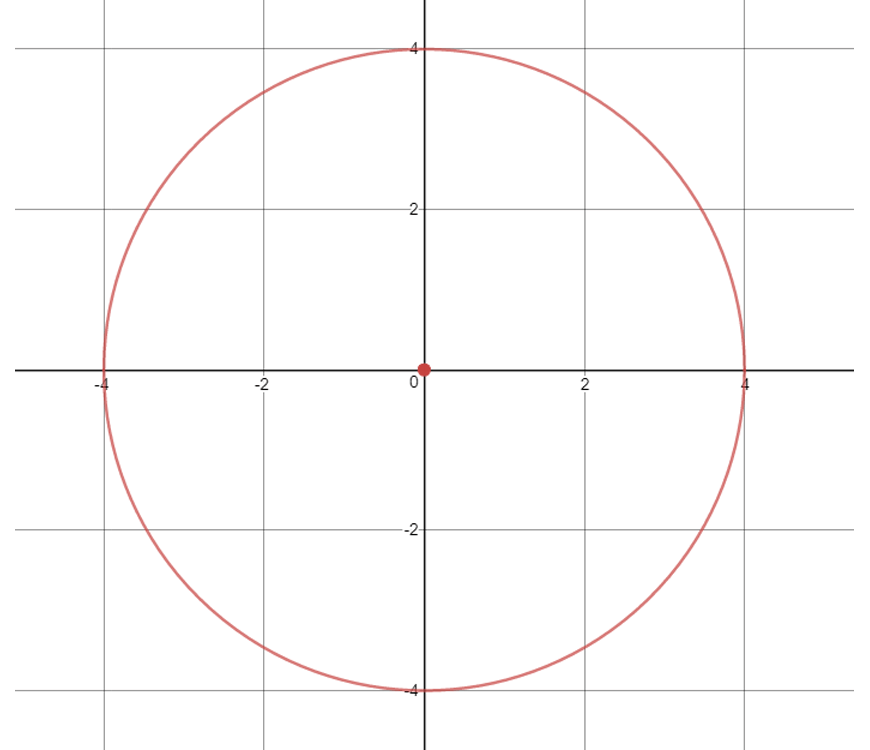It has a centre at the origin $$(0,0)$$, with a radius of $$4$$.

Notice how we needed to square root the 16 in the equation to get the actual radius length of $$4$$.

### $$(x-k)^2+(y-h)^2=r^2$$ | Moving The Centre

Circles can also have a centre which is not the origin, dictated by subtracting a constant inside the squares.

In this general case, the centre would be at $$(k,h)$$. It is very important to note the minus signs in the general case, and in normal questions we should flip the sign of the constant to find the coordinates of the centre.

For example, let’s investigate the circle $$(x-4)^2+(y+3)^2=4$$.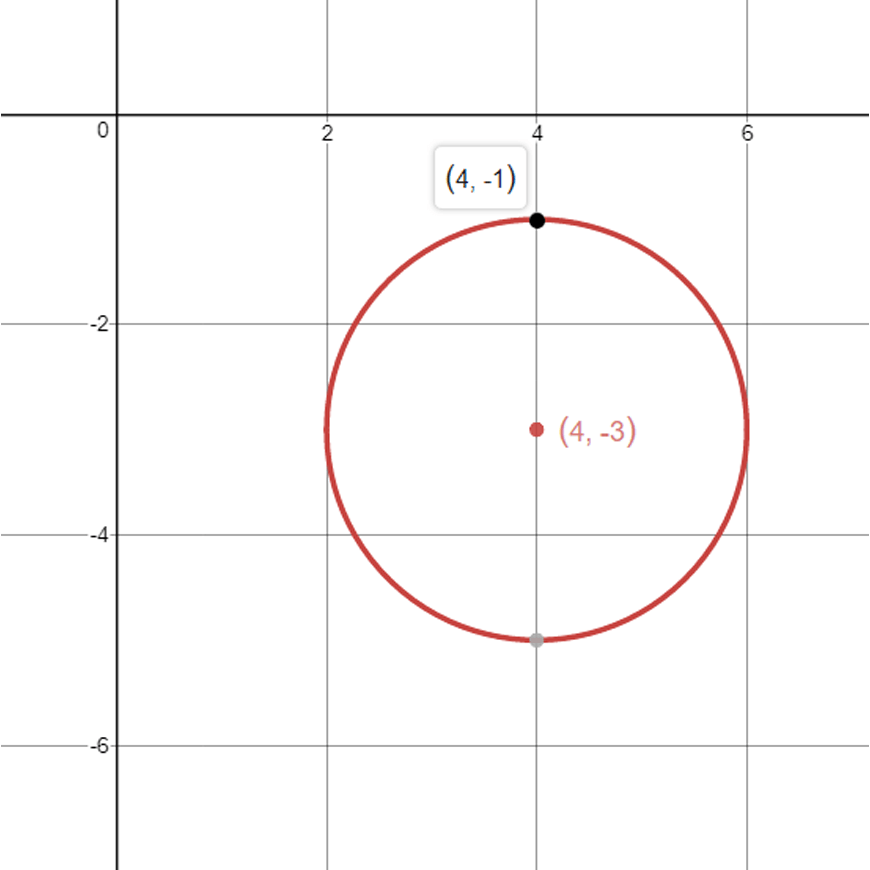This circle has a centre at $$(4,-3)$$, with a radius $$2$$ (remember to square root the $$4$$ first!).

Notice that the x-coordinate of the centre $$(4)$$ has the opposite sign as the constant in the expression $$(x-4)^2$$.

Similarly, the $$y$$-coordinate of the centre $$(-3)$$ has the opposite sign as the constant in the expression $$(y+3)^2$$.

Knowing the centre and the radius of the circle, it is easy to sketch it on the plane.

## Checkpoint Questions

Sketch the following:

1. $$y=-3x^2$$

2. $$y=(x-3)^3$$

3. $$y=\frac{-1}{(x+2)}+2$$

4. $$y=\frac{1}{(5-x)}+\frac{2}{3}$$

5. $$(x-5)^2+y^2=9$$

6. $$(x+10)^2+(y-10)^2=100$$

7. $$y=(4-x)^2-4$$

8. $$y=\frac{1}{(2x-4)}$$

9. $$y=\frac{4}{5}-x^3$$

10. $$y=\frac{(x+5)}{(x+2)}$$ (Challenge!)

## Solutions

1. A simple negative parabola, with vertex $$(0,0)$$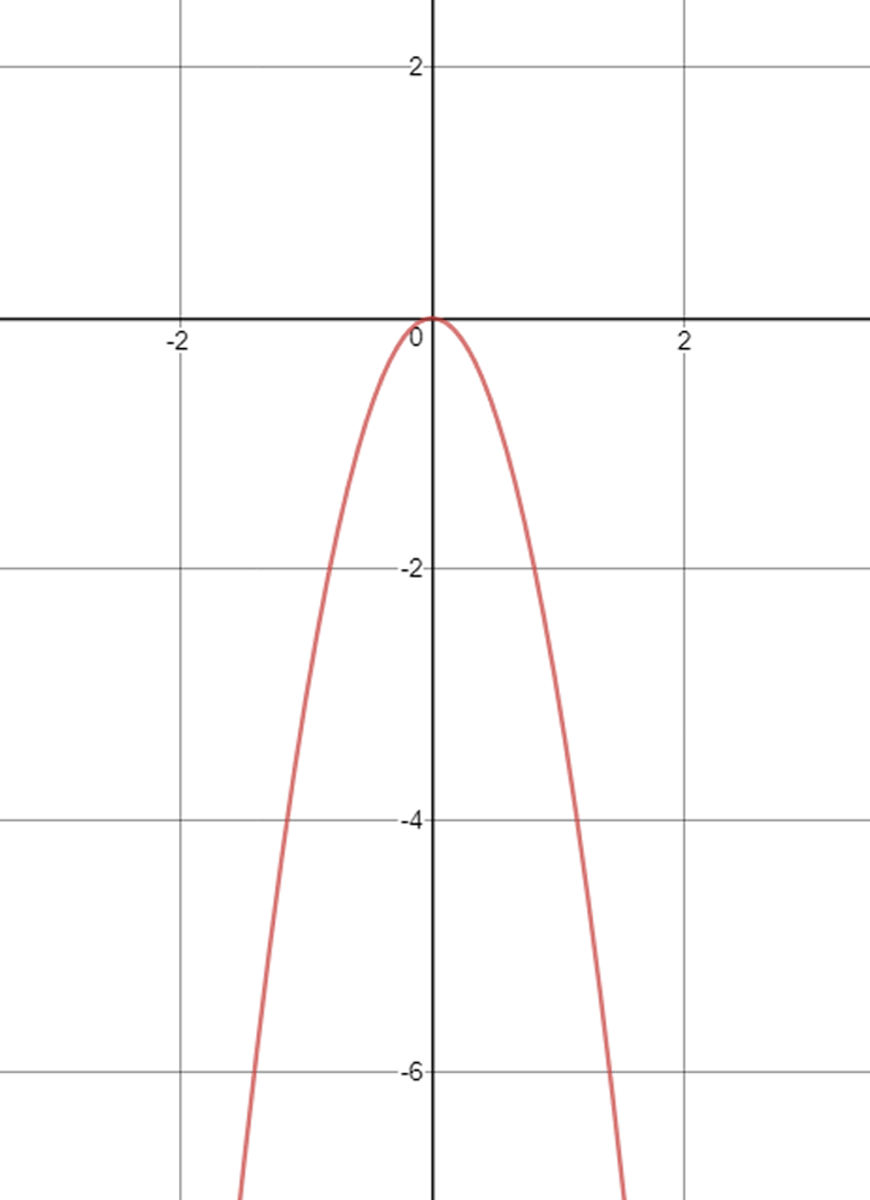2. A positive cubic, with POI shifted to the right by $$3$$ units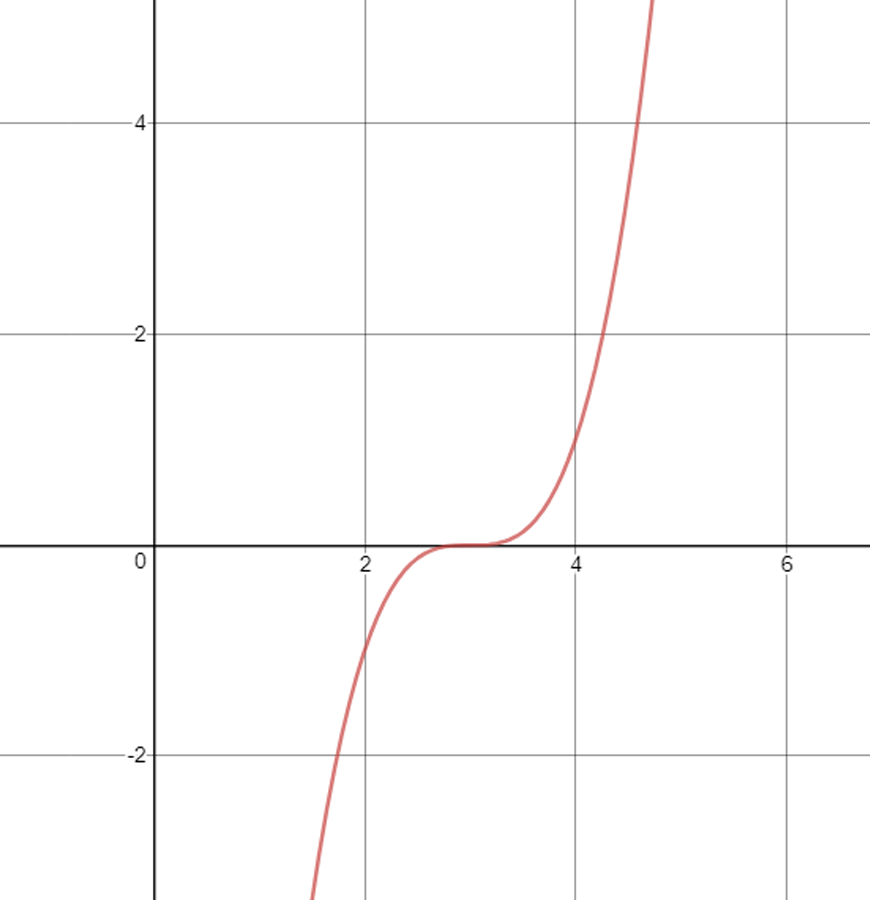3. A negative hyperbola, shifted to the left by $$2$$ and up by $$2$$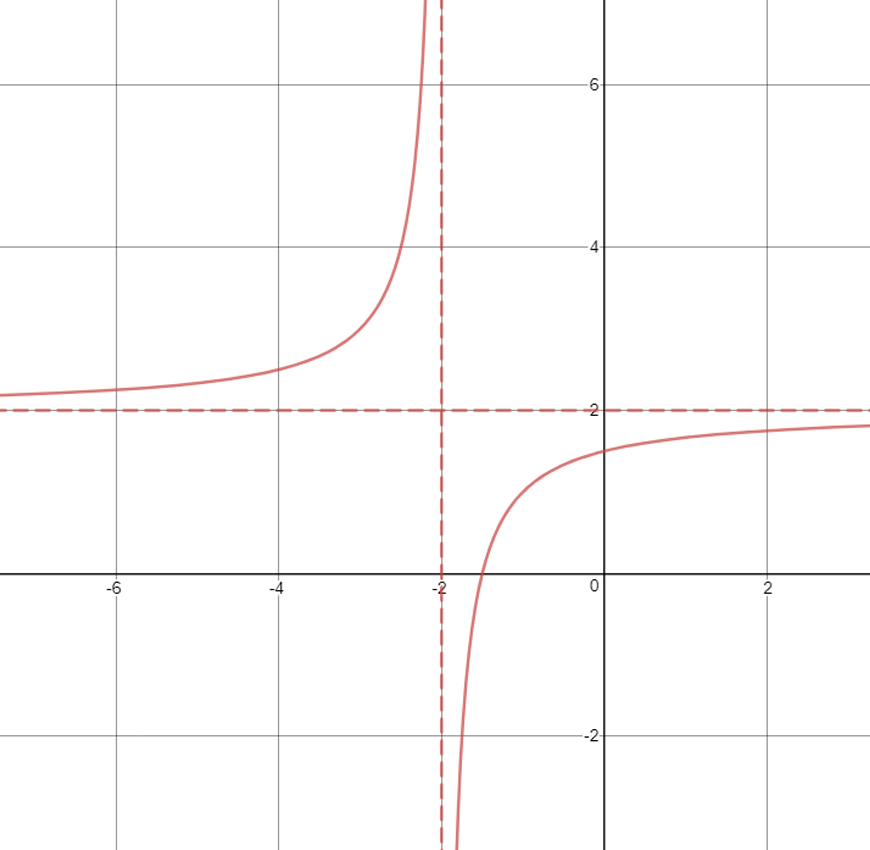4. Since there is a minus sign in front of the $$x$$, we should first factorise out a $$-1$$ from the denominator, and rewrite it as $$y=\frac{-1}{(x-5)}+\frac{2}{3}$$.

Now we can see that it is a negative hyperbola, shifted right by $$5$$ and up by $$\frac{2}{3}$$.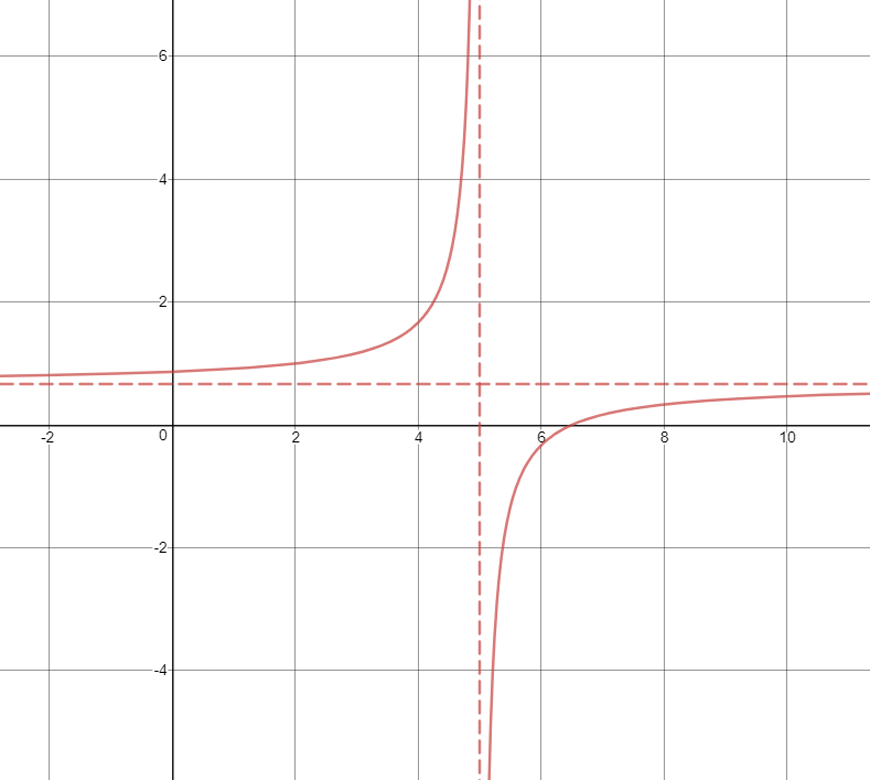5. A circle with centre $$(5,0)$$ and radius $$3$$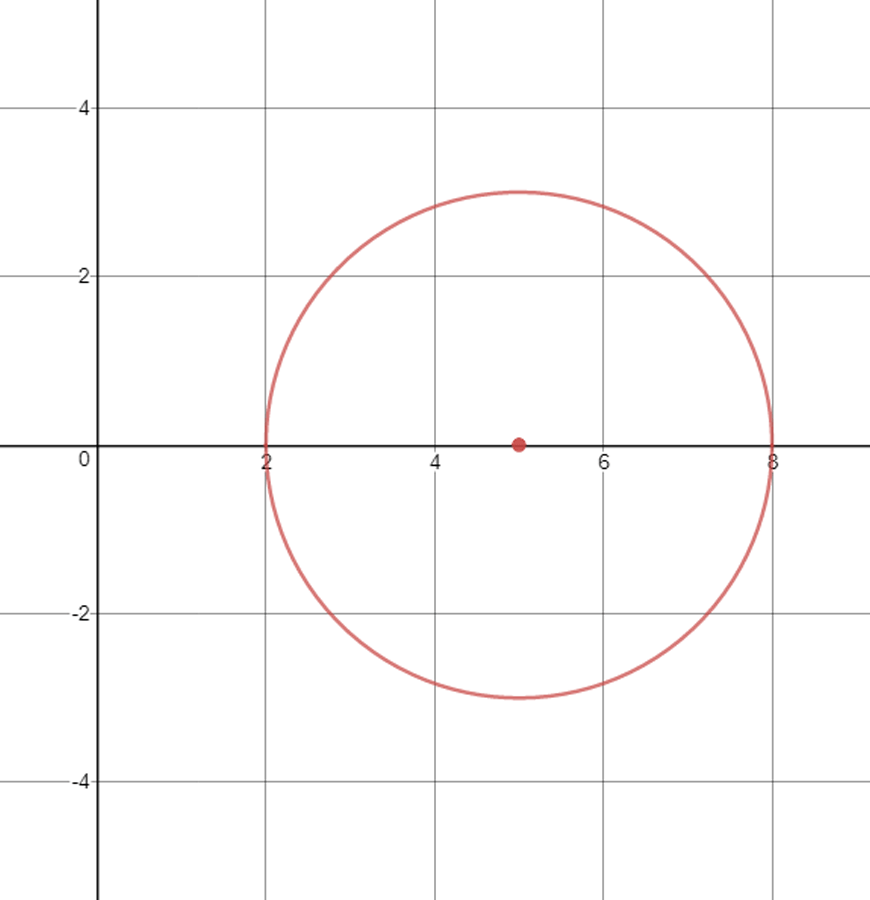6. A circle with centre $$(-10,10)$$ and radius $$10$$. Notice how the circle should just barely touch the $$x$$ and $$y$$ axes at –$$10$$ and $$10$$ respectively.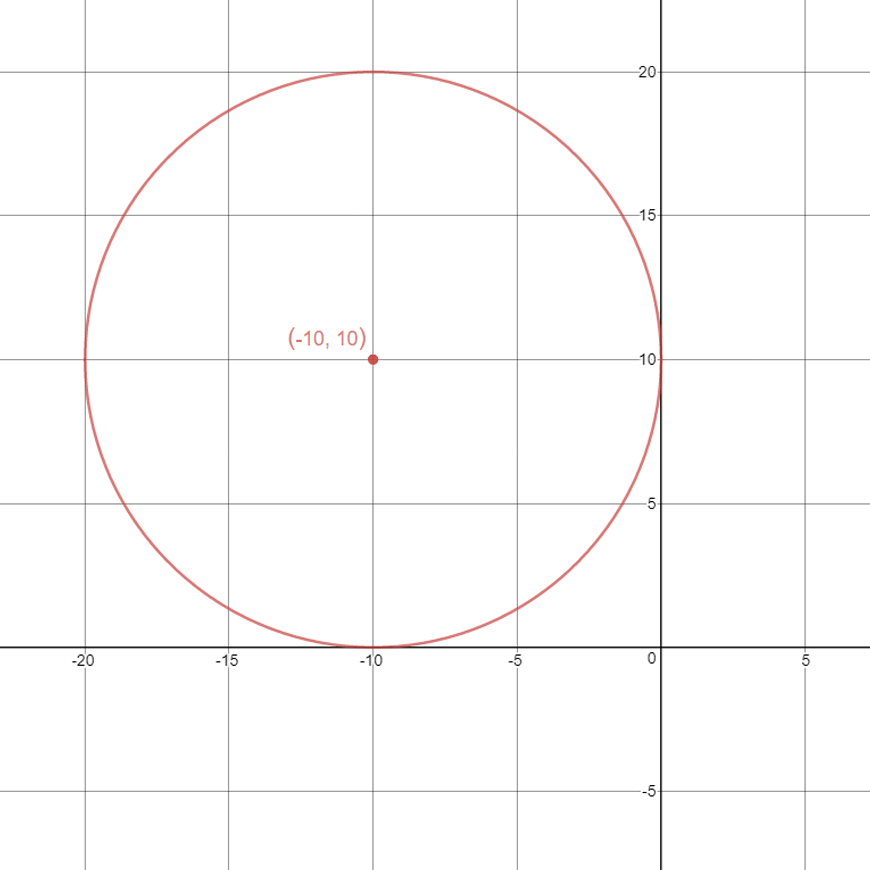7. There is a negative in front of the $$x$$, so we should take out a $$-1$$.

However, since that factorised $$-1$$ is also squared, it just becomes $$1$$ again.

Notice how $$(4-x)^2$$ is the same as $$(x-4)^2$$.

So the final equation should be $$y=(x-4)^2-4$$.

This is a positive parabola, shifted right by $$4$$ and down by $$4$$.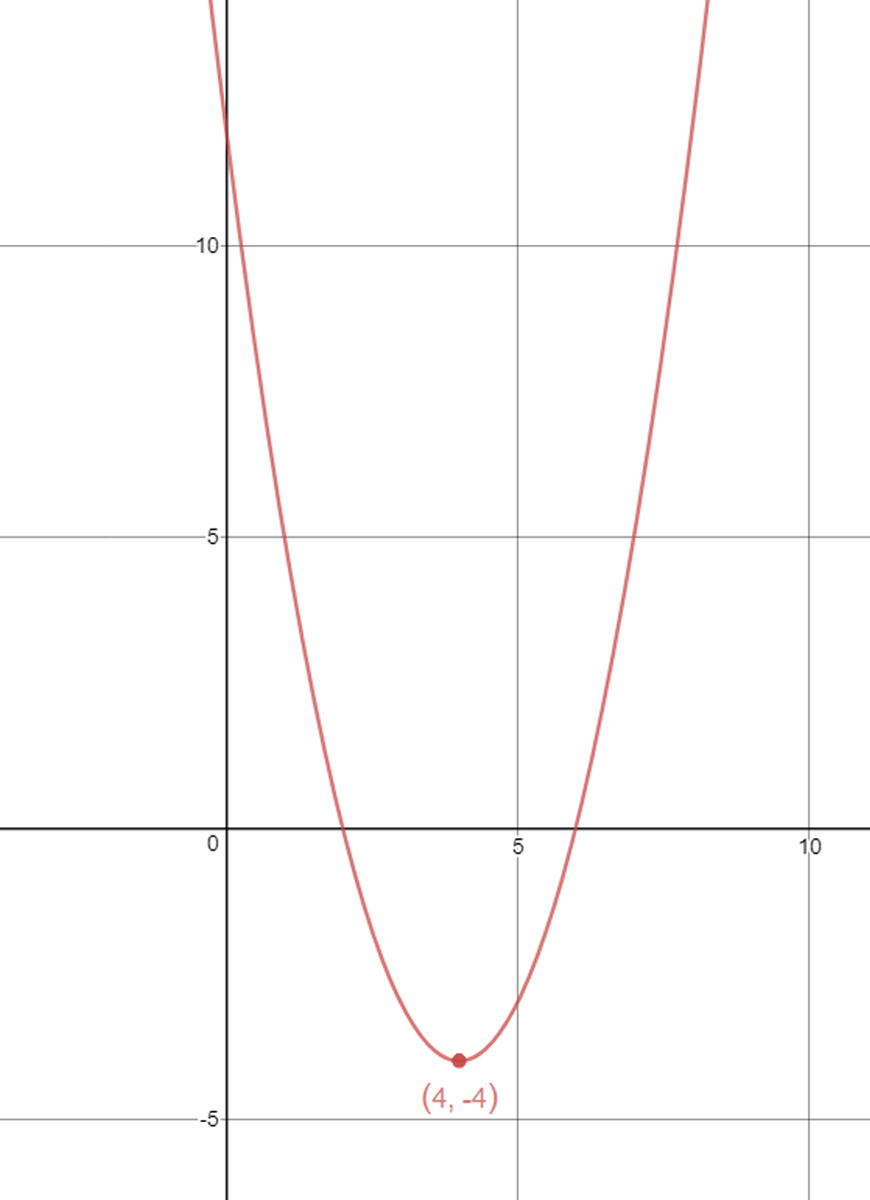8. Since there is a $$2$$ in front of the $$x$$, we should first factorise $$2$$ from the denominator.

Notice this is the same as factorising $$\frac{1}{2}$$ from the entire fraction.

So the equation becomes $$y=\frac{1}{2}\times \frac{1}{(x-2)}$$.

This is just a scaled positive hyperbola, shifted to the right by $$2$$.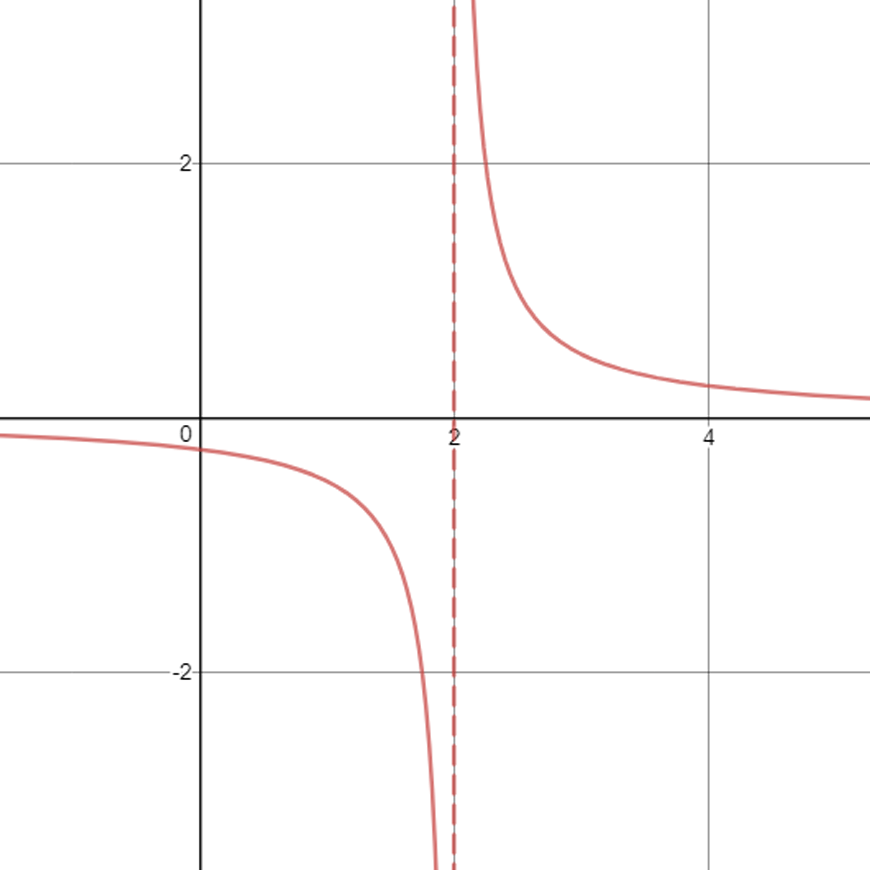Notice how the scaling factor of $$\frac{1}{2}$$ doesn’t change the shape of the graph at all.

9. Let’s first rearrange the equation so the $$x^3$$ term comes first, followed by any constants.

So we have $$-x^3+\frac{4}{5}$$.

This is simply a negative cubic, shifted up by $$\frac{4}{5}$$ units.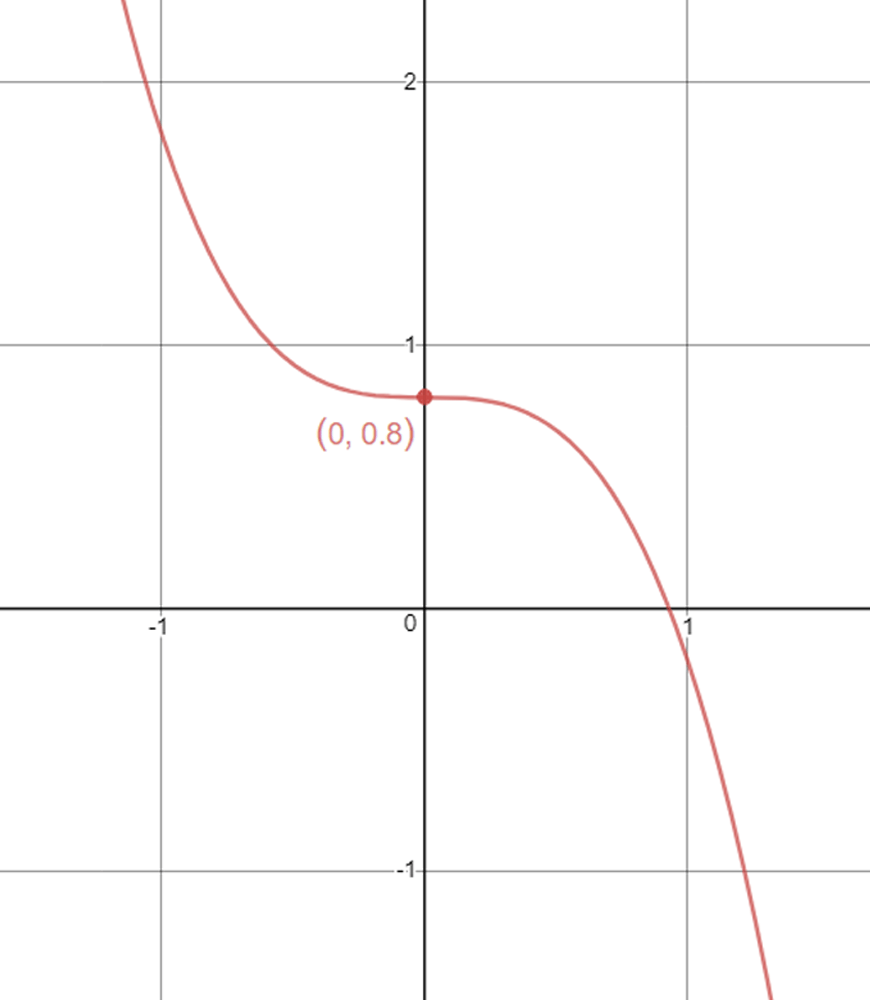10.  At first, this doesn’t really look like any of the forms we have dealt with.

However, notice how the $$5$$ in the numerator can be broken up into $$2+3$$.

So we have:

$$y=\frac{(x+2+3)}{(x+2)}$$

We can now split the fraction into two, taking $$x+2$$ as one numerator and $$3$$ as the other. $$y=\frac{(x+2)}{(x+2)}+\frac{3}{(x+2)}$$

Clearly, the first term just cancels to become $$1$$.

So our final equation is: $$y=1+\frac{3}{(x+2)}$$

This is simply a (scaled) hyperbola, shifted left by $$2$$ and up by $$1$$.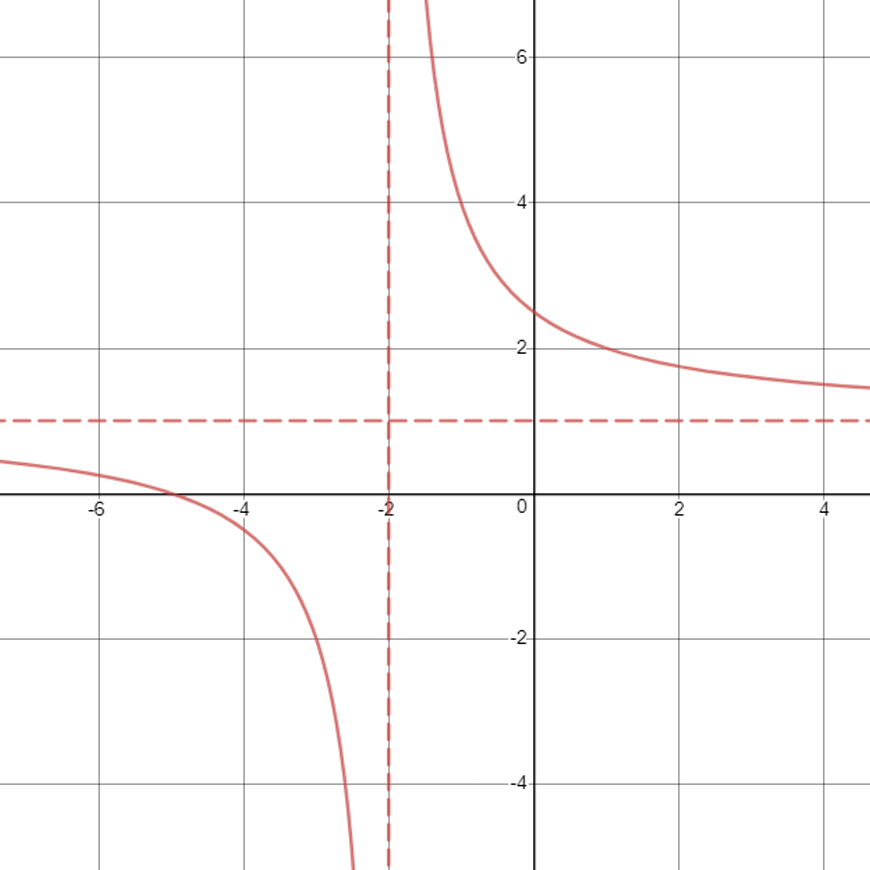## Summary

Curve sketching is an extremely underrated skill that – if mastered- can make many topics in senior mathematics much easier.

Students who have a good grasp of how algebraic equations can relate to the coordinate plane, tend to do well in future topics, such as calculus.

This subject guide is just the beginning of the skills students will learn in curve sketching, as their knowledge will build from here all the way until they finish their HSC.

We hope that you’ve learnt something new from this subject guide, so get out there and ace mathematics!

## Want to ace your next Maths exam?## Build a solid understanding in functions

In our next article, we explain the foundations of functions. Functions are one of the important foundations for Year 11 and 12 Maths.

© Matrix Education and www.matrix.edu.au, 2021. Unauthorised use and/or duplication of this material without express and written permission from this site’s author and/or owner is strictly prohibited. Excerpts and links may be used, provided that full and clear credit is given to Matrix Education and www.matrix.edu.au with appropriate and specific direction to the original content.